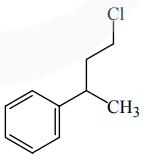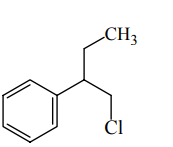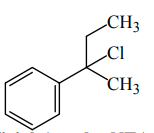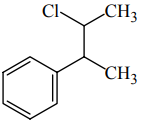# Reaction of Grignard reagent,`
Question:

Reaction of Grignard reagent, $\mathrm{C}_{2} \mathrm{H}_{5} \mathrm{MgBr}$ with $\mathrm{C}_{8} \mathrm{H}_{8} \mathrm{O}$ followed by hydrolysis gives compound "A" which reacts instantly with Lucas reagent to give compound $\mathrm{B}, \mathrm{C}_{10} \mathrm{H}_{13} \mathrm{Cl}$. The Compound B is :

1.2.3.4.Correct Option: , 3

Solution:

Official Ans. by NTA (3)